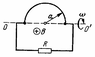# Average power dissipation for induced current

auctor

## Homework Statement

A wire shaped as a semi-circle of radius a rotates about the axis OO’ with a constant angular velocity ω in a uniform magnetic field with induction B (attached figure). The plane of the rectangular loop is perpendicular to the magnetic field direction. The total resistance of the circuit is R. Neglecting effects of the magnetic field that is generated by the current in the circuit, find the mean thermal power dissipated during one rotation period.

## Homework Equations

Emf: ε=-dΦ/dt
Flux: dΦ=B⋅dS
Angle: ϑ=ωt
Current: I=ε/R
Power: P=εI
0 sin2(x)dx = 1/2

## The Attempt at a Solution

The area of the projection of the loop onto the direction perpendicular to the magnetic field changes according to S=Srectangle+πa2/2 cos(ϑ)=Srectangle+πa2/2 cos(ωt)
The magnetic induction isn't changing.
The flux as a function of time is then Φ=B(Srectangle+πa2/2 cos(ωt))
The emf is ε=-dΦ/dt = ωBπa2/2 sin(ωt)
The current is I=ωBπa2/(2R) sin(ωt)
The instantaneous power is P=[ωBπa2]2/(4R) sin2(ωt)
The average power is P=[ωBπa2]2/(8R)

However, the answer the book gives is P=[ωBπa2]2/(2R). This result would correspond to a variation of the projection area S=Srectangle+πa2 cos(ϑ). Not sure why this would be the case...

#### Attachments

•Screen Shot 2018-04-02 at 11.49.59 AM.png
5.7 KB · Views: 480
Last edited: